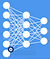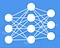# Avoid Overfitting in Deep Learning

In this blog, we will write about regularization. we will discuss its purpose and how it works.

If there is one thing that jeopardizes a perfect Neural Network that would be overfitting. Overfitting refers to situations where the model has fit the training data so well that the model captures the noise and random fluctuations.

I assume we already know about using a validation set and early stopping in order to prevent overfitting from happening. Unfortunately, I have to say that these approaches are not 100% reliable. There may be certain situations where the validation loss stays the same or increases marginally but the model still in the process of obtaining valuable insights from the training set. Therefore, we don’t want the early stopping to kick in at this moment. In theory that can be countered by fiddling with the “patience” parameter of the early stopping callback. Unfortunately, we are not always aware of what a value should be or whether a particular value is any good.

Overall, it is a good idea to have fundamentally different tools that combat overfitting. logically, different tools are useful in different situations and nothing stops us from employing them all.

now, in what other way we can prevent overfitting? actually, there is a clever answer to this question. Since overfitting happens when the model learns too much we can combat this by interfering with the model’s ability to learn. In other words, we decrease the model’s capacity. At first, it seems like a counterproductive measure. After all, the whole purpose of machine learning is to make a machine learn! but of course, the devil is in the details and the extent to which we prevent the model from learning is the important part.

How do we decrease the model’s capacity? there are various ways to achieve that: probably the simplest one is to make the model itself simpler. In fact, if you are employing a linear regression, no one will ever mention overfitting to you as the linear regression is very simple by definition. now, downscaling the complexity to linear regression is a bit drastic; There must be some middle ground right? that’s what regularization is all about. In a nutshell, regularization is a method used to reduce the model’s capacity to learn. More precisely, it restricts the capacity of complex models and encourages simpler representations of the solution.

Technically speaking, regularization consists of including additional factors to the loss function to change the behavior of the training process.

New Loss = Old Loss + Regularization factor

Since the backpropagation is solely based on the loss, changing the loss affects the optimization of weights. What does that mean for an actual Neural Network? well, we can incentivize or penalize behavior. For instance, we can increase the loss when an undesired event occurs subsequently the model will adjust itself to not trigger that event as much. Inversely, we can decrease the loss in a situation where we want to encourage the model to follow the desired pattern.

Going back to our goal we want to inhibit the capacity of the network. So, how can we represent the complexity of a network with numbers that can be added to the loss function? Intuitively, if a model has learned very simple representations of the solution we would expect a significant portion of parameters to be zero or close to zero. We can convince ourselves of the validity of the statement by looking at what actually happens if a weight set to zero.weights of a neuron set to zero

Nullifying this parameter detaches the neuron from the network as if it doesn’t exist. Do that for a large number of neurons at the graph of this network starts to look a lot more simplistic.The simplistic look of the network after detaching neurons

Conversely, if the model is complex most if not all the weights would be non-zero numbers. Therefore, we can conclude that the complexity or capacity of our model can be described by the value of the weights. The exact combination of these values determines the types of regularization.

M.Sc in Information Technology

## More from Ali Mahzoon

M.Sc in Information Technology